# Texas Go Math Grade 5 Lesson 2.3 Answer Key Division with 2-Digit Divisors

Refer to our Texas Go Math Grade 5 Answer Key Pdf to score good marks in the exams. Test yourself by practicing the problems from Texas Go Math Grade 5 Lesson 2.3 Answer Key Division with 2-Digit Divisors.

## Texas Go Math Grade 5 Lesson 2.3 Answer Key Division with 2-Digit Divisors

Investigate

Materials: base-ten blocks

There are 156 students in the Carville Middle School chorus. The music director wants the students to stand with 12 students in each row for the next concert. How many rows will there be?

A. Use base-ten blocks to model the dividend, 156.

B. Place 2 tens below the hundred to form a rectangle. How many groups of 12 does the rectangle show? How much of the dividend is not shown in this rectangle?
When we divide 120 into blocks of ten, there will be 10 blocks of 12.
The dividend that is not shown in the rectangle will be: 36

C. Combine the remaining tens and ones into as many groups of 12 as possible. How many groups of 12 are there?
From part (B),
We can observe that
There are remaining 36 blocks after we placed 120 blocks
Now,
We can arrange 36 blocks into 3 groups of 12
Hence, from the above,
We can conclude that
There are 3 groups of 12

D. Place these groups of 12 on the right side of the rectangle to make a larger rectangle.
From part A,
There are 10 groups of 12
From part B,
There are 3 groups of 12
So,
The total number of groups of 12 = 10 + 3
= 13 groups of 12

E. The final rectangle shows groups of 12.

So,
There will be 13 rows of 12 students.

Draw Conclusions

Question 1.
Explain why you still need to make groups of 12 after Step B.
In Step B,
We made 10 groups of 12.
But,
There are 36 remaining blocks
So,
We have to find whether we can make groups of 12 with 36 or not
Hence,
We will still need to make groups of 12 after Step B

Question 2.
Describe how you can use base-ten blocks to find the quotient 176 ÷ 16.
The given division expression is: 176 ÷ 16
Now,
Step A:
Divide 176 into 16 base-ten blocks
So,
The 16 ten-base blocks will be equal to 160 and the remainder will be 16
Step B:
Find out whether 16 will be divided into groups of 16
Step C:
We can make 1 group of 16 blocks
Hence, from the above,
We can conclude that
The quotient of 176 ÷ 16 by using the base-ten blocks is: 11

The two sets of groups of 12 that you found in the Investigate are partial quotients. First, you found 10 groups of 12, and then you found 3 more groups of 12. Sometimes you may need to regroup before you can show a partial quotient.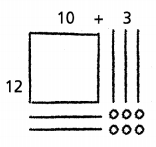You can use a quick picture to record the partial products.

Divide. 180 ÷ 15

MODEL Use base-ten blocks.

STEP 1: Model the dividend, 180, as 1 hundred 8 tens.
Model the first partial quotient by making a rectangle with the hundred and 5 tens. In the Record, cross out the hundred and tens you use.
So,
The rectangle shows 10 groups of 15.

Regroup 1 ten as 10 ones. In the Record, cross out the regrouped ten.

There are now 25 tens and 5 ones.

STEP 3: Decide how many additional groups of 15 can be made with the remaining tens and ones. The number of groups is the second partial quotient.

Make your rectangle larger by including these groups of 15. In the Record, cross out the tens and ones you use.
So,
There are now 12 groups of 15.

RECORD Use quick pictures.Draw the first partial quotient.Draw the first and second partial quotients.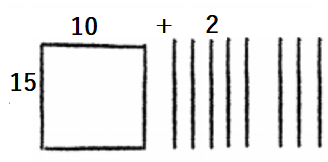So,
180 ÷ 15 is 12

Math Talk
Mathematical Processes

Explain how your model shows the quotient.
When we draw a model for the given division expression,
We will draw in the form of partial products
So,
When we add the Partial numbers that are divided, the result will be given the value of the quotient for the given division expression

Share and Show

Use the quick picture to divide.

Question 1.
143 ÷ 13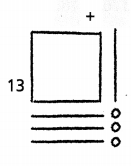The given division expression is: 143 ÷ 13
Now,
By using the model,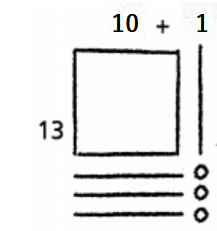Now,
From the above model,
We can observe that
The quotient for the given division expression = 10 + 1
= 11
Hence, from the above,
We can conclude that
The quotient for the given division expression is: 11

Use base-ten blocks or a quick picture to divide.

Question 2.
168 ÷ 12
The given division expression is: 168 ÷ 12
Now,
By using the model,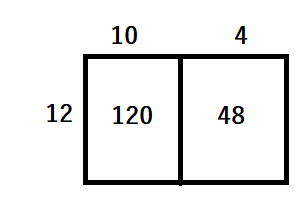Now,
From the above model,
We can observe that
The quotient for the given division expression = 10 + 4
= 14
Hence, from the above,
We can conclude that
The quotient for the given division expression is: 14

Question 3.
187 ÷ 11
The given division expression is: 187 ÷ 11
Now,
By using the model,Now,
From the above model,
We can observe that
The quotient for the given division expression = 10 + 7
= 17
Hence, from the above,
We can conclude that
The quotient for the given division expression is: 17

Question 4.
182 ÷ 13
The given division expression is: 182 ÷ 13
Now,
By using the model,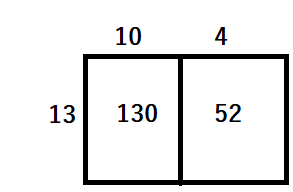Now,
From the above model,
We can observe that
The quotient for the given division expression = 10 + 4
= 14
Hence, from the above,
We can conclude that
The quotient for the given division expression is: 14

Question 5.
228 ÷ 12
The given division expression is: 228 ÷ 12
Now,
By using the model,Now,
From the above model,
We can observe that
The quotient for the given division expression = 10 + 9
= 19
Hence, from the above,
We can conclude that
The quotient for the given division expression is: 19

Problem Solving

The Pony Express used men riding horses to deliver mail between St. Joseph, Missouri, and Sacramento, California, from April 1860 to October 1861. The trail between the cities was approximately 2,000 miles long.

Question 6.
H.O.T. Multi-Step Two Pony Express riders each rode part of a 176-mile trip. Each rider rode the same number of miles. They changed horses every 11 miles. How many horses did each rider use?It is given that
The Pony Express used men riding horses to deliver mail between St. Joseph, Missouri, and Sacramento, California, from April 1860 to October 1861. The trail between the cities was approximately 2,000 miles long.
It is also given that
Two Pony Express riders each rode part of a 176-mile trip. Each rider rode the same number of miles. They changed horses every 11 miles.
So,
According to the given information,
The number of horses did each rider used = (The distance traveled by the two Pony Expresses) ÷ (The number of miles the Pony Expresses changed the horses)
= 176 ÷ 11
Now,
By using the Long Division,Hence, from the above,
We can conclude that
The number of horses did each rider used throughout the total distance is: 16 horses

Question 7.
Suppose a Pony Express rider was paid $192 for 12 weeks of work. If he was paid the same amount each week, how much was he paid for each week of work? Answer: It is given that A Pony Express rider was paid$192 for 12 weeks of work and he was paid the same amount each week
So,
According to the given information,
The amount he paid for each week = (The total amount a rider paid for some weeks) ÷ (The number of weeks)
= $192 ÷ 12 Now, By using the Long Division,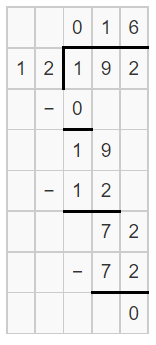Hence, from the above, We can conclude that The amount a Pony Express Rider paid for each week of work is:$16

Question 8.
H.O.T. Multi-Step It took 19 Pony Express riders a total of 11 days 21 hours to ride from St. Joseph to Sacramento. If they all rode the same number of hours, how many hours did each rider ride?
It is given that
It took 19 Pony Express riders a total of 11 days 21 hours to ride from St. Joseph to Sacramento and they all rode the same number of hours
Now,
We know that,
1 day = 24 hours
So,
The total number of hours in 11 days = 24 × 11
= 264 hours
So,
The total number of hours did 19 riders took to travel from St. Joseph to Sacramento = 264 + 21
= 285 hours
Now,
The number of hours did each rider took to travel from St. Joseph to sacramento = 285 ÷ 19
Now,
By using the Long Division,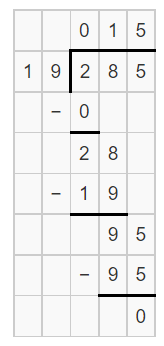Hence, from the above,
We can conclude that
The number of hours did each rider took to travel from St. Joseph to sacramento is: 15 hours

Question 9.
Use Diagrams A rover brings 144 rock samples back from Mars. The samples will be divided among 12 teams of scientists for analysis. Which quick picture shows the correct division of the samples between the 12 teams?It is given that
A rover brings 144 rock samples back from Mars. The samples will be divided among 12 teams of scientists for analysis
So,
The correct division of the samples between 12 teams = 144 ÷ 12
Hence, from the above,
We can conclude that
The picture that shws the correct division of the samples between 12 teams is:Question 10.
Analyze The Mars rover is going to travel across a crater that is 360 cm (about 12 ft) in diameter. The rover must stop every 15 cm to recharge its batteries. How many times will the rover recharge while it crosses the crater?
(A) 24
(B) 15
(C) 30
(D) 25
It is given that
The Mars rover is going to travel across a crater that is 360 cm (about 12 ft) in diameter. The rover must stop every 15 cm to recharge its batteries
Now,
According to the given information,
The number of times will the rover recharge while it crosses the crater = (The distance Mars rover is going to travel) ÷ (The time took by rover torecharge its batteries)
= 360 ÷ 15
Now,
By using the long Division,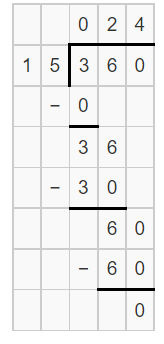Hence, from the above,
We can conclude that
The number of times will the rover recharge while it crosses the crater is: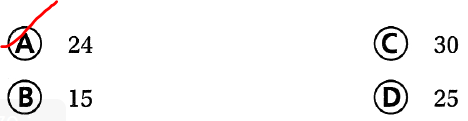Question 11.
Multi-Step On Monday, the rover traveled 330 cm. On Tuesday, it traveled 180 cm. If the rover stopped every 15 cm to recharge, how many more times did it need to recharge on Monday than on Tuesday?
(A) 24
(B) 22
(C) 12
(D) 10
It is given that
On Monday, the rover traveled 330 cm. On Tuesday, it traveled 180 cm. If the rover stopped every 15 cm to recharge
Now,
According to the given information,
The difference between the number of times the rover recharge on Monday and Tuesday = (330 ÷ 15) – (180 ÷ 15)
= 22 – 12
= 10 times
Hence, from the above,
We can conclude that
The difference between the number of times the rover recharge on Monday and Tuesday is:Texas Test Prep

Question 12.
Analyze Three Pony Express riders each rode part of a 252-mile trip. If they used a total of 18 horses and rode each horse the same number of miles, how many miles did they ride before replacing each horse?
(A) 54 miles
(B) 14 miles
(C) 84 miles
(D) 42 miles
It is given that
Three Pony Express riders each rode part of a 252-mile trip. If they used a total of 18 horses and rode each horse the same number of miles
Now,
According to the given information,
The number of miles the three Pony Express riders rode before replacing each horse = 252 ÷ 18
Now,
By using the Long Division,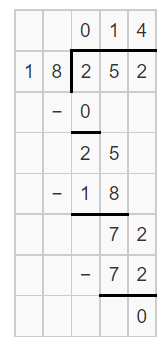Hence, from the above,
We can conclude that
The number of miles the three Pony Express riders rode before replacing each horse is: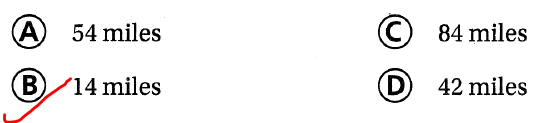### Texas Go Math Grade 5 Lesson 2.3 Homework and Practice Answer Key

Use base-ten blocks or a quick picture to divide.

Question 1.
180 ÷ 12
The given division expression is: 180 ÷ 12
Now,
By using the model,Now,
From the above model,
We can observe that
The quotient for the given division expression = 10 + 5
= 15
Hence, from the above,
We can conclude that
The quotient for the given division expression is: 15

Question 2.
216 ÷ 18
The given division expression is: 216 ÷ 18
Now,
By using the Model,Now,
From the above model,
We can observe that
The quotient for the given division expression = 10 + 2
= 12
Hence, from the above,
We can conclude that
The quotient for the given division expression is: 12

Question 3.
224 ÷ 16
The given division expression is: 224 ÷ 16
Now,
By using the Model,Now,
From the above model,
We can observe that
The quotient for the given division expression = 10 + 4
= 14
Hence, from the above,
We can conclude that
The quotient for the given division expression is: 14

Question 4.
176 ÷ 16
The given division expression is: 176 ÷ 16
Now,
By using the Model,Now,
From the above model,
We can observe that
The quotient for the given division expression = 10 + 1
= 11
Hence, from the above,
We can conclude that
The quotient for the given division expression is: 11

Problem Solving

Question 5.
Ryan read 145 pages of his book. If he read 29 pages every night, how many nights did it take him to complete the book?
It is given that
Ryan read 145 pages of his book and he read 29 pages every night
Now,
According to the given information,
The number of nights did it take Ryan to complete the book = 145 ÷ 29
Now,
By using the Long Division,Hence, from the above,
We can conclude that
The number of nights did it take Ryan to complete the book is: 5 nights

Question 6.
Mrs. McDonald passed out 189 photos that she had taken during the school year. If each of her 21 students received the same number of photos, how many did each receive?
It is given that
Mrs. McDonald passed out 189 photos that she had taken during the school year and each of her 21 students received the same number of photos
Now,
According to the given information,
The number of photos did Mrs.McDonald received = 189 ÷ 21
Now,
By using the Long Division,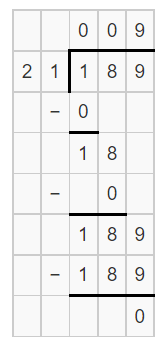Hence, from the above,
We can conclude that
The number of photos did Mrs.McDonald received is: 9 photos

Lesson Check

Question 7.
Susanna made a total of $132 for the school fundraiser. She sold pizza combo meals for$12 each. How many combo meals did Susanna sell?
(A) 10
(B) 11
(C) 13
(D) 12
It is given that
Susanna made a total of $132 for the school fundraiser. She sold pizza combo meals for$12 each.
Now,
According to the given information,
The number of combo meals did Susanna sold = $132 ÷$12
Now,
By using the Long Division,Hence, from the above,
We can conclude that
The number of combo meals did Susanna sold is: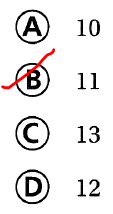Question 8.
Carlos’ horse ran around the track a total of 336 times. If the horse ran 21 laps each day, how many days did it take the horse to complete all of the laps?
(A) 20
(B) 16
(C) 18
(D) 12
It is given that
Carlos’ horse ran around the track a total of 336 times. If the horse ran 21 laps each day
Now,
According to the given information,
The number of days did it took for the horse to complete all of the laps = 336 ÷ 21
Now,
By using the Long Division,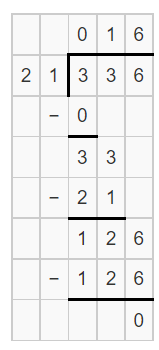Hence, from the above,
We can conclude that
The number of days did it took for the horse to complete all of the laps is: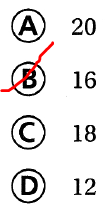Question 9.
Milos rode his bicycle in a marathon for 154 miles. He took a rest stop every 22 miles. How many rest stops did Milos take during the marathon?
(A) 8
(B) 7
(C) 10
(D) 9
It is given that
Milos rode his bicycle in a marathon for 154 miles. He took a rest stop every 22 miles
Now,
According to the given information,
The number of rest stops did Milos took during the marathon = 154 ÷ 22
Now,
By using the Long Division,Hence, from the above,
We can conclude that
The number of rest stops did Milos took during the marathon is:Question 10.
There are 325 books on the library wall. There are 13 rows of books, each with an equal number of books. How many books are in each row?
(A) 28
(B) 21
(C) 25
(D) 19
It is given that
There are 325 books on the library wall. There are 13 rows of books, each with an equal number of books
Now,
According to the given information,
The number of books in each row = 325 ÷ 13
Now,
By using the Long Division,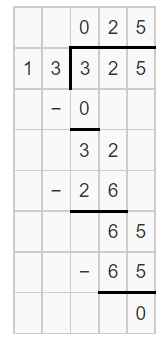Hence, from the above,
We can conclude that
The number of books in each row is:Question 11.
Multi-Step Lilly was paid $308 for 14 weeks of work. If she was paid the same amount each week, how much did she earn after 2 weeks? (A)$38
(B) $28 (C)$22
(D) $44 Answer: It is given that Lilly was paid$308 for 14 weeks of work and she was paid the same amount each week
Now,
According to the given information,
The amount of money earned by Lilly each week = $308 ÷ 14 Now, By using the Long Division,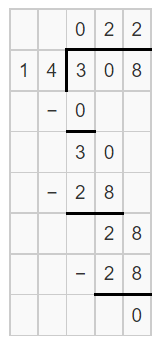So, The amount of money earned by Lilly in 2 weeks = 2 ×$22
= \$44
Hence, from the above,
We can conclude that
The amount of money earned by Lilly in 2 weeks is: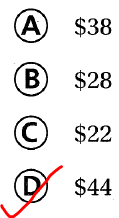Question 12.
Multi-Step Manny drove 910 miles during a two-week period. If he drove the same number of miles each day, how many miles had he driven after 5 days?
(A) 182 miles
(B) 250 miles
(C) 650 miles
(D) 325 miles
It is given that
Manny drove 910 miles during a two-week period and he drove the same number of miles each day
Now,
We know that,
1 week = 7 days
Now,
According to the given information,
The number of miles did Manny rode each day = 910 ÷ 14
Now,
By using the Long Division,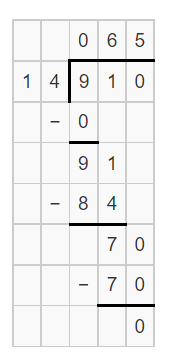So,
The number of miles did Manny rode in 5 days = 5 × 65
= 325 miles
Hence, from the above,
We can conclude that
The number of miles did Manny rode in 5 days is:Scroll to Top